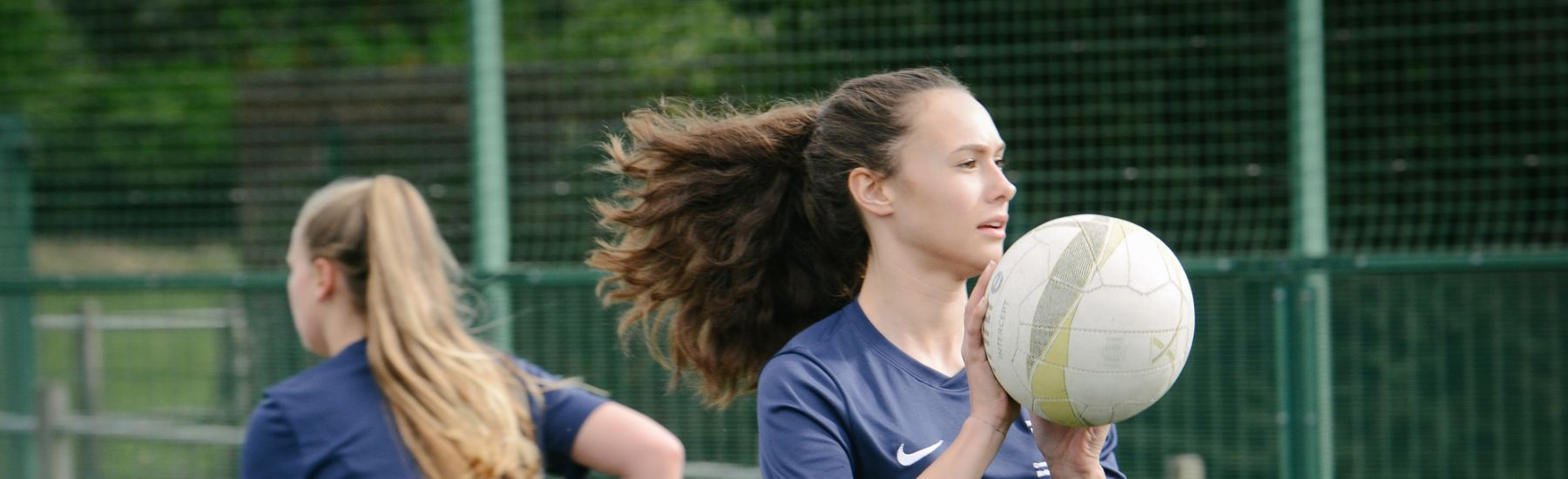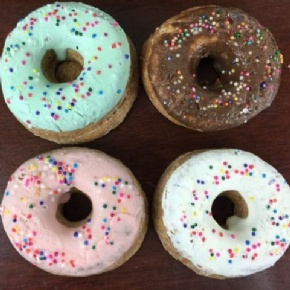# Ivybridge Community College

•# Is A Doughnut Mathematically Identical to a Mug?In January four Year 7 students attended a Mathematics Masterclass on Polygons, Polyhedrons, Flexagons and Topology.

In January four Year 7 students attended a Mathematics Masterclass on Polygons, Polyhedrons, Flexagons and Topology at Plymouth University to prove that a doughnut is mathematically identical to a mug.  Students were involved in a variety of workshops in which they investigated different aspects of Topology, which is the study of surfaces.

Students discovered that knowing that internal angles of a triangle always add up to 180° is very important when it comes to finding the internal angles of lots of other regular polygons.  Having talked about regular polygons they discovered that all polygons can be divided into triangles to make finding their internal angles easier.The latter part of the day was spent looking at three dimensional polygons (polyhedra) which introduced them into the world of Euler`s formula.  Although the mathematics involved in proofing the formula was a bit difficult for the students they were told that they would probably meet it again if they go on to do A Level Mathematics.

Thanks go to Mrs Medlicott who accompanied the students for the day.